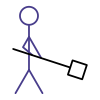## docs for Kojo

These pages describe the Gaming facility within Kojo.This section of the website is currently under development.

For now, here are some simple animations leading up to a Lunar Lander game:

Note - computer programs in Kojo are written using the Scala programming language. You will need to know the Basics of Scala to understand the programs below.

You can copy the programs below and paste them into Kojo to run them.

### Bouncing shape

``````clear()
drawStage(ColorMaker.black)
val cb = canvasBounds

val pic = Picture {
setFillColor(red)
repeat(4) {
forward(40)
right(90)
}
}
pic.setPosition(cb.x + 20, cb.y + 20)
var vel = Vector2D(2, 10)

draw(pic)

animate {
pic.translate(vel)
if (pic.collidesWith(stageBorder)) {
vel = bouncePicVectorOffStage(pic, vel)
}
}
``````

### Bouncing shape with gravity

``````clear()
drawStage(ColorMaker.black)
val cb = canvasBounds

class Ball {
val pic = Picture.rectangle(40, 40)
pic.setFillColor(red)
pic.setPosition(cb.x + 20, cb.y + 20)
var vel = Vector2D(2, 10)
val gravity = Vector2D(0, -0.2)

def draw() {
pic.draw()
}

def step() {
vel = vel + gravity
pic.translate(vel)
if (pic.collidesWith(stageBorder)) {
vel = bouncePicVectorOffStage(pic, vel)
}
}
}

val ball = new Ball()
ball.draw()

animate {
ball.step()
}
``````

### Bouncing sprite with gravity

``````clear()
drawStage(ColorMaker.black)
val cb = canvasBounds

class Ball {
val pic1 = Picture.image("/media/flappy-ball/ballwing1.png")
val pic2 = Picture.image("/media/flappy-ball/ballwing2.png")
val pic = picBatch(
pic1,
pic2
)
pic.setPosition(cb.x + 20, cb.y + 20)
var vel = Vector2D(2, 10)
val gravity = Vector2D(0, -0.2)

def draw() {
pic.draw()
}

def step() {
vel = vel + gravity
pic.translate(vel)
if (pic.collidesWith(stageBorder)) {
vel = bouncePicVectorOffStage(pic, vel)
}
pic.showNext(150)
}
}

val ball = new Ball()
ball.draw()

animate {
ball.step()
}
``````

### Lunar Lander

``````cleari()
drawStage(ColorMaker.hsl(240, 0.20, 0.16))

class Lander {
val body = fillColor(red) -> Picture.rectangle(40, 70)
val thruster = fillColor(orange) -> Picture.rectangle(20, 35)
thruster.setPosition(body.position.x + 10, body.position.y - 20)

val gravity = Vector2D(0, -0.1)
var velocity = Vector2D(0, 0)
var thrust = Vector2D(0, 0)

def draw() {
body.draw()
thruster.draw()
thruster.invisible()
}

def step() {
if (isKeyPressed(Kc.VK_UP)) {
inThrust()
}
else {
noThrust()
}
velocity = velocity + gravity
velocity = velocity + thrust

body.translate(velocity)
thruster.setPosition(body.position.x + 10, body.position.y - 20)

if (body.collidesWith(stageBorder)) {
velocity = bouncePicVectorOffStage(body, velocity)
}
}

def inThrust() {
thrust = new Vector2D(0, 1)
thruster.visible()
}

def noThrust() {
thrust = new Vector2D(0, 0)
thruster.invisible()
}
}

class Moon {
val pic = trans(370, -350) -> Picture {
setPenColor(cm.lightBlue)
setFillColor(cm.darkGray)
left(45)
left(90, 500)
}

def draw() {
pic.draw()
}

def check(l: Lander) {
if (l.body.collidesWith(pic)) {
if (l.velocity.y.abs > 3) {
drawCenteredMessage("You Lose", red, 39)
}
else {
drawCenteredMessage("You Win", green, 30)
}
stopAnimation()
}
}

}

val l = new Lander()
l.draw()

val m = new Moon()
m.draw()

animate {
l.step()
m.check(l)
}
activateCanvas()
``````

### Tic-tac-toe

``````cleari()
val cb = canvasBounds
setBackground(black)
disablePanAndZoom()
val len = 100

class Board(bx: Double, by: Double) {
val margin = 20
val len2 = len - 2 * margin
val lineWidth = 8

def background() {
setPenColor(null)
setFillColor(black)
val mgn = lineWidth / 2
setPosition(mgn, mgn)
repeat(4) {
forward(len - 2 * mgn)
right(90)
}
}

def cross = Picture {
background()
setPenThickness(lineWidth)
setPenColor(ColorMaker.hsl(200, 1.00, 0.50))
setPosition(margin, margin)
lineTo(len - margin, len - margin)
setPosition(len - margin, margin)
lineTo(margin, len - margin)
}

def o = Picture {
background()
setPenThickness(lineWidth)
setPenColor(ColorMaker.hsl(120, 0.86, 0.64))
setPosition(len / 2, margin)
left(360, len2 / 2)
}

def blank = Picture {
background()
}

val lines = Picture {
setPenThickness(lineWidth)
repeatFor(1 to 2) { n =>
setPosition(len * n, 0)
lineTo(len * n, 3 * len)
}
repeatFor(1 to 2) { n =>
setPosition(0, len * n)
lineTo(3 * len, len * n)
}
}

val pics = Array.ofDim[Picture](3, 3)
val state = Array.ofDim[Int](3, 3)

var nextCross = true
var done = false

def show() {
lines.setPosition(bx, by)
draw(lines)
repeatFor(0 until 3) { x =>
repeatFor(0 until 3) { y =>
val pic = blank
pic.setPosition(bx + x * len, by + y * len)
draw(pic)
pic.onMouseClick { (_, _) =>
if (!done) {
val newPic = if (nextCross) {
val np = cross
np.setPosition(pic.position)
state(x)(y) = 2
np
}
else {
val np = o
np.setPosition(pic.position)
state(x)(y) = 1
np
}
nextCross = !nextCross
pics(x)(y) = newPic
draw(newPic)
pic.erase()
checkWin()
if (!done) {
checkDraw()
}
}
}
pics(x)(y) = pic
state(x)(y) = 0
}
}
}

def column(x: Int) = state(x)
def row(y: Int) = Array(state(0)(y), state(1)(y), state(2)(y))
def diagonal1 = Array(state(0)(0), state(1)(1), state(2)(2))
def diagonal2 = Array(state(0)(2), state(1)(1), state(2)(0))

def checkWinFor(n: Int): Boolean = {
var win = false
val target = Array(n, n, n)
repeatFor(0 until 3) { x =>
win = column(x).sameElements(target)
if (win) {
return true
}
}

repeatFor(0 until 3) { y =>
win = row(y).sameElements(target)
if (win) {
return true
}
}
win = diagonal1.sameElements(target)
if (win) {
return true
}
win = diagonal2.sameElements(target)
win
}

def gameOver(msg: String) {
val pmsg = Picture {
setPenFontSize(80)
setPenColor(white)
write(msg)
}
val pic = picColCentered(pmsg, Picture.vgap(cb.height - 100))
drawCentered(pic)
done = true
}

def checkWin() {
if (checkWinFor(1)) {
gameOver("O Won")
}
else if (checkWinFor(2)) {
gameOver("X Won")
}
}

def checkDraw() {
var filled = true
repeatFor(0 until 3) { x =>
repeatFor(0 until 3) { y =>
if (state(x)(y) == 0) {
filled = false
}
}
}
if (filled) {
done = true
gameOver("It's a Draw")
}
}
}

val boardSize = len * 3

setup {
val b = new Board(cb.x + (cb.width - boardSize) / 2, cb.y + (cb.height - boardSize) / 2)
b.show()
}
``````

Contribute Content